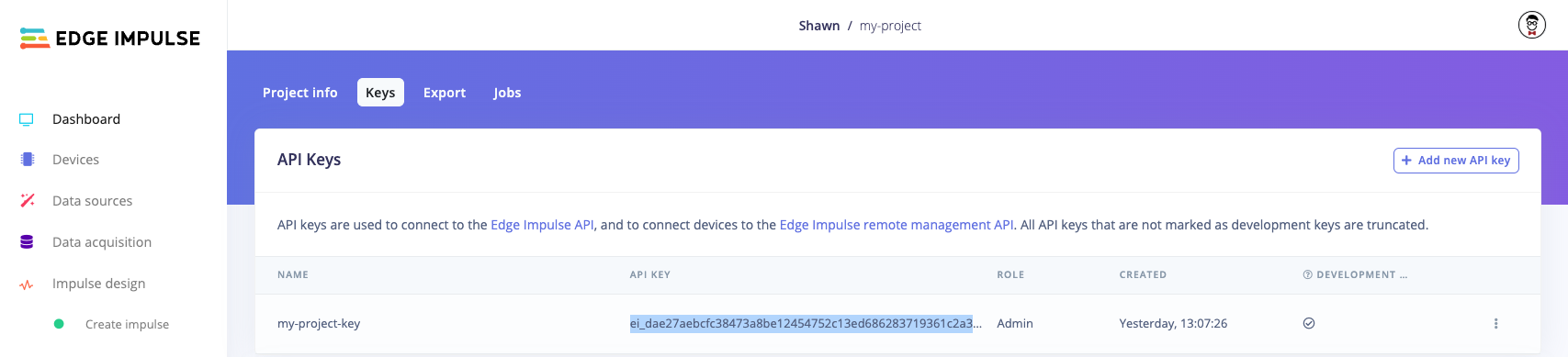# Using the Edge Impulse Python SDK with TensorFlow and Keras

TensorFlow is an open source library for training machine learning models. Keras is an open source Python library that makes creating neural networks in TensorFlow much easier. We use these two libraries together to very quickly train a model to identify handwritten digits. From there, we use the Edge Impulse Python SDK library to profile the model to see how inference will perform on a target edge device. Then, we use the SDK again to convert our trained model to a C++ library that can be deployed to an edge hardware platform, such as a microcontroller.
Follow the code below to see how to train a simple machine learning model and deploy it to a C++ library using Edge Impulse.
# If you have not done so already, install the following dependencies
!python -m pip install tensorflow==2.12.0 edgeimpulse
from tensorflow import keras
import edgeimpulse as ei
You will need to obtain an API key from an Edge Impulse project. Log into edgeimpulse.com and create a new project. Open the project, navigate to Dashboard and click on the Keys tab to view your API keys. Double-click on the API key to highlight it, right-click, and select Copy.Copy API key from Edge Impulse project
Note that you do not actually need to use the project in the Edge Impulse Studio. We just need the API Key.
Paste that API key string in the `ei.API_KEY` value in the following cell:
# Settings
ei.API_KEY = "ei_dae2..." # Change this to your Edge Impulse API key
labels = ["0", "1", "2", "3", "4", "5", "6", "7", "8", "9"]
num_classes = len(labels)
deploy_filename = "my_model_cpp.zip"

## Train a machine learning model

We want to create a classifier that can uniquely identify handwritten digits. To start, we will use TensorFlow and Keras to train a very simple convolutional neural network (CNN) on the classic MNIST dataset, which consists of handwritten digits from 0 to 9.
(x_train, y_train), (x_test, y_test) = keras.datasets.mnist.load_data()
x_train = keras.utils.normalize(x_train, axis=1)
x_test = keras.utils.normalize(x_test, axis=1)
y_train = keras.utils.to_categorical(y_train, num_classes)
y_test = keras.utils.to_categorical(y_test, num_classes)
input_shape = x_train.shape
# Build the model
model = keras.Sequential([
keras.layers.Flatten(),
keras.layers.Dense(32, activation='relu', input_shape=input_shape),
keras.layers.Dense(num_classes, activation='softmax')
])
# Compile the model
loss='categorical_crossentropy',
metrics=['accuracy'])
# Train the model
model.fit(x_train,
y_train,
epochs=5)
# Evaluate model on test set
score = model.evaluate(x_test, y_test, verbose=0)
print(f"Test loss: {score}")
print(f"Test accuracy: {score}")

To start, we need to list the possible target devices we can use for profiling. We need to pick from this list.
# List the available profile target devices
ei.model.list_profile_devices()
You should see a list printed such as:
['alif-he',
'alif-hp',
'arduino-nano-33-ble',
'arduino-nicla-vision',
'portenta-h7',
'brainchip-akd1000',
'cortex-m4f-80mhz',
'cortex-m7-216mhz',
...
'ti-tda4vm']
A common option is the `cortex-m4f-80mhz`, as this is a relatively low-power microcontroller family. From there, we can use the Edge Impulse Python SDK to generate a profile for your model to ensure it fits on your target hardware and meets your timing requirements.
# Estimate the RAM, ROM, and inference time for our model on the target hardware family
try:
profile = ei.model.profile(model=model,
device='cortex-m4f-80mhz')
print(profile.summary())
except Exception as e:
print(f"Could not profile: {e}")

Once you are happy with the performance of the model, you can deploy it to a number of possible hardware targets. To see the available hardware targets, run the following:
# List the available profile target devices
ei.model.list_deployment_targets()
You should see a list printed such as:
['zip',
'arduino',
'tinkergen',
'cubemx',
'wasm',
...
'runner-linux-aarch64-tda4vm']
The most generic target is to download a .zip file that holds a C++ library containing the inference runtime and your trained model, so we choose `'zip'` from the above list. To do that, we first need to create a Classification object which contains our label strings (and other optional information about the model). These strings will be added to the C++ library metadata so you can access them in your edge application.
Note that instead of writing the raw bytes to a file, you can also specify an `output_directory` argument in the `.deploy()` function. Your deployment file(s) will be downloaded to that directory.
# Set model information, such as your list of labels
model_output_type = ei.model.output_type.Classification(labels=labels)
# Create C++ library with trained model
deploy_bytes = None
try:
deploy_bytes = ei.model.deploy(model=model,
model_output_type=model_output_type,
deploy_target='zip')
except Exception as e:
print(f"Could not deploy: {e}")